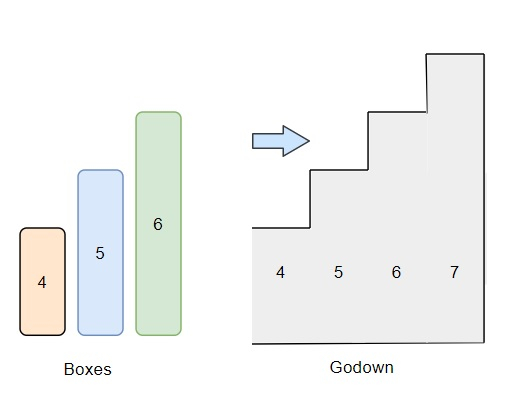# Program to find out the number of boxes to be put into the godown in Python

Suppose, we have two arrays containing integers. One list contains the height of some unit width boxes and another array contains the height of rooms in the godown. The rooms are numbered 0...n, and the height of the rooms is provided in their respective indexes in the array godown. We have to find out the number of boxes that can be pushed into the godown. a few things have to be kept in mind,

• The boxes can’t be put one on another.

• The order of the boxes can be changed.

• The boxes are put into the godown from left to right only.

If a box is taller than the height of the room, then the box along with all the boxes to its right cannot be pushed into the godown.

So, if the input is like boxes = [4,5,6], godown = [4, 5, 6, 7], then the output will be 1 Only one box can be inserted. The first room is of size 4 and the rest cannot be pushed into the godown because the boxes have to be pushed through the first room and its length is smaller than the other boxes.To solve this, we will follow these steps −

• sort the list boxes

• curmin := a new list containing the first element of godown

• cm := curmin

• for i in range 1 to size of godown, do

• cur := godown[i]

• if cur < cm, then

• cm := cur

• insert cm at the end of curmin

• i := 0

• j := size of godown -1

• r := 0

• while j >= 0 and i < size of boxes, do

• if curmin[j] >= boxes[i], then

• i := i + 1

• r := r + 1

• j := j - 1

• return r

## Example (Python)

Let us see the following implementation to get better understanding −

Live Demo

def solve(boxes, godown):
boxes.sort()
curmin = [godown]
cm = curmin
for i in range(1, len(godown)):
cur = godown[i]
if cur < cm:
cm = cur
curmin.append(cm)
i,j = 0, len(godown)-1
r = 0
while j >= 0 and i < len(boxes):
if curmin[j] >= boxes[i]:
i += 1
r += 1
j -= 1
return r

print(solve([4,5,6], [4, 5, 6, 7]))

## Input

[4,5,6], [4, 5, 6, 7]

## Output

1